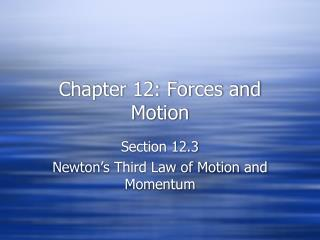DownloadDownload PresentationChapter 12: Forces and Motion

# Chapter 12: Forces and Motion

Download Presentation## Chapter 12: Forces and Motion

- - - - - - - - - - - - - - - - - - - - - - - - - - - E N D - - - - - - - - - - - - - - - - - - - - - - - - - - -
##### Presentation Transcript

1. Chapter 12: Forces and Motion Section 12.3 Newton’s Third Law of Motion and Momentum

2. Newton’s Third Law of Motion • States that whenever one object exerts a force on a second object, the second object exerts an equal and opposite force on the first object.

3. Action and Reaction Forces • These forces that are produced by the two objects are action and reaction forces. • Sometimes action and reaction forces result in motion – sometimes they don’t. • Action and reaction forces do NOT cancel themselves out.

4. Momentum • Product of an object’s mass and its velocity • An object at rest has a momentum of 0. • Momentum = Mass x Velocity • Measured in kilogram-meters/second • p = mv

5. Conservation of Momentum • Momentum is conserved in a closed system. • A closed system of objects means that there are no outside forces or objects acting on the objects in the system. • In a closed system, the loss of momentum by one object equals the gain of momentum by the second object.